# Mean velocity for turbulent velocity distribution in pipe.

SherlockOhms

## Homework Statement

Calculate the mean velocity (um) for turbulent velocity distribution in a pipe.

## Homework Equations

um = Q/A
u = umax(y/R)1/7 (Gives velocity at a specified point in cross section of the pipe.
R = pipe diameter. y = distance from boundary layer. umax = distance at centre.

## The Attempt at a Solution

I'll post a diagram of what I've done so far. As you can see, I've split the cross section of the pipe into rings of flow of thickness dr and radius r. So, dA = 2(pi)(r)(dr). dQ = u(dA)
Integrate in the limit 0 to R. Q = ∫ umax(y/R)1/7(2(pi)(r)(dr)).
How do I integrate the above? That's what's really confusing me. Is u constant and so can it factor out of the integral? The y term is really throwing me off. Thanks.

SherlockOhms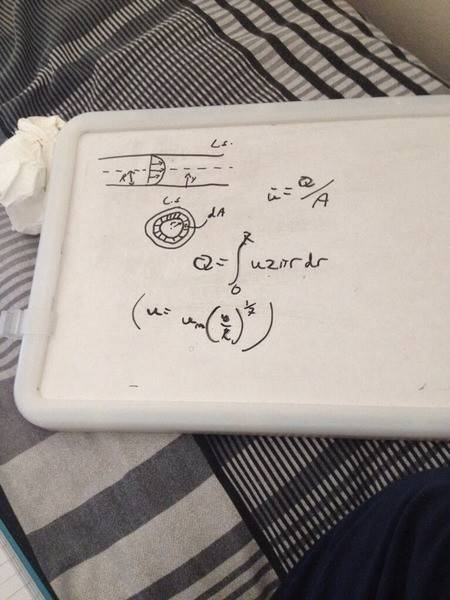Homework Helper
Gold Member
Dearly Missed
Now, u_max(x) is certainly a constant on a CROSS-section.

Assume the boundary layer has thickness d(x), within it, the velocity is so small compared to the flow in the middle, that it can be regarded as 0. (Alternatively, it should fuse together with the established boundary layer velocity profile!)

Thus, we have that 0<=r<=R-d(x), rather than 0<=r<=R

Furthermore, y equals 0 at the edge of the boundary layer, and R-d(x) along the center line.

Thus, we have: y=R-d(x)-r

SherlockOhms
I thin he mentioned that we can ignore the thickness of the boundary layer for this calculation. I'll have to double check that though. Thanks a million for that! So, I've now got Q = umax∫((R-r)/R)1/7(2(pi)(r)dr fro 0 to R. How do I actually integrate this? Haven't done proper calculus in awhile. Should I be using a substitution? Like, u = (R-r)/R.

Homework Helper
Gold Member
Dearly Missed
Yes, that will be a good substitution.(And, as I wrote it, it really does have best meaning if d(x) is NEGLIGIBLE relative to R)
Since d(x) only appears together with R, we may discard it, both in the integrand and in the integration limit.

SherlockOhms
So, I've done the integration but I'm fairly sure it's incorrect. I'll post my step by step solution below. The lecturer gave us the final solution, i.e the value of Q/(pi)(R2). I'll show that value too in the attachment. Can you see where I may have gone wrong? Thanks again.

SherlockOhms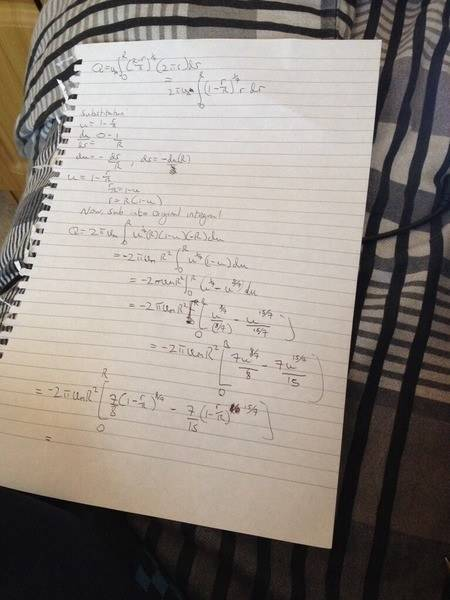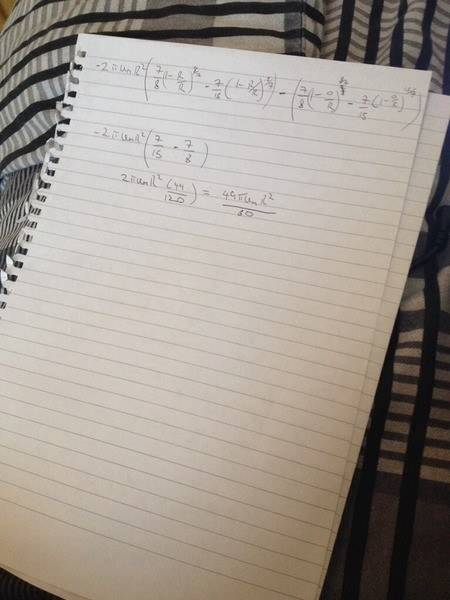SherlockOhms
This is the value of the mean velocity, i.e. Q/A. As you can see my equation for Q, divided by A does not equal this.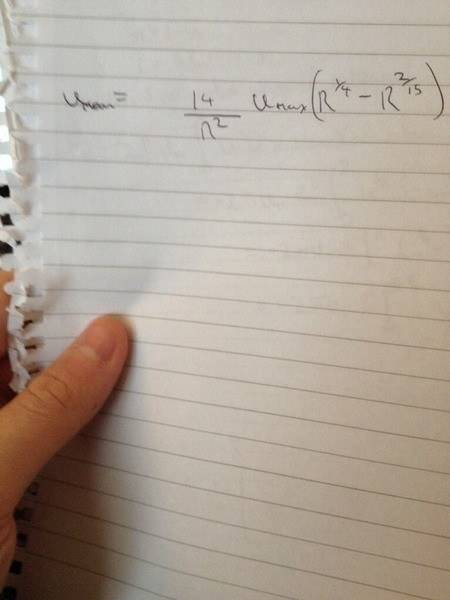SherlockOhms
I'm now thinking that I've actually solved that integral correctly to get Q = ((49)(pi)(umax)(R^2))/60. However if I divide this by the Area, I get u(mean) = (49)(pi)(umax)/60. Which is completely independent of R. This hardly makes sense, does it? Is the a chance that this is correct and the lecturers solution is incorrect? I've also checked the integral in Mathematica and it works out.

SherlockOhms
49(umax)/60. Apologies, the pis cancel.

Homework Helper
Gold Member
Dearly Missed
If Mathematica gets the same result as you, (and that I got as well), I'm sure it's correct.
That doesn't mean, however, that you have given all relevant information or typed the problem in here correctly.

I strongly suggest you go back to your lecture notes and see if there is something you have overlooked.

SherlockOhms
Yup. Thanks for that. Just went back and looked at the exact notes he gave us today. The picture I've attached below is word for word and diagram for diagram what he gave us. Everything there was given and the rest (pretty much just the integral) was left up to us. Have I missed something?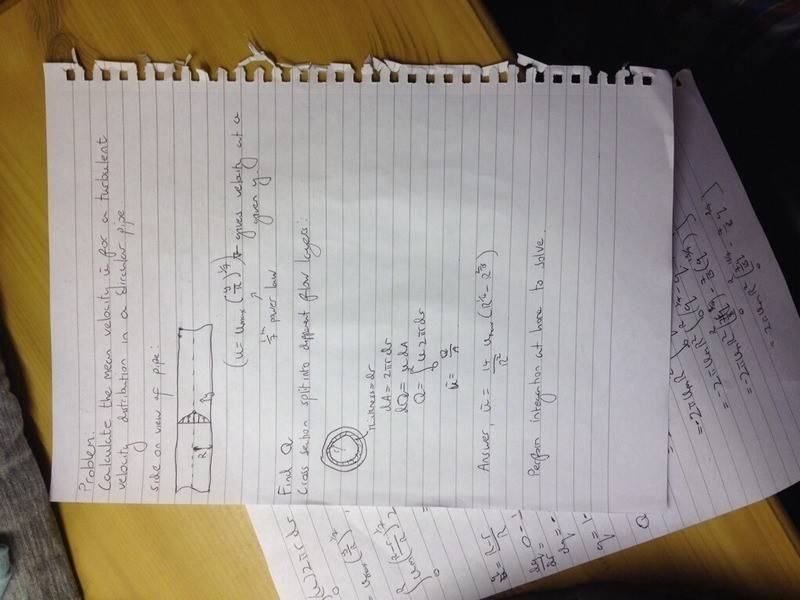Homework Helper
Gold Member
Dearly Missed
Hmm..it doesn't seem so.

SherlockOhms
Strange. I guess I'll just have to ask the lecturer himself at some stage. Thanks for all the help.

Mentor
Why do you find it so unusual that the mean velocity is 49/60 of the maximum velocity, or that the maximum velocity is 60/49 of the mean velocity? In laminar flow, the maximum velocity is 2 times the mean velocity, but in turbulent flow, the velocity profile is nearly flat, so that the ratio is only about 1.2.

SherlockOhms
Well, I'm new to Fluid kinematic and didn't know that at all. I just though that, intuitively, the mean velocity would depend on the radius of the pipe in some way. But here (assuming I haven't made any mistakes) it's completely independent of the pipe radius. There was no real physics behind that assumption. It was just something that I thought made sense to me at the time.